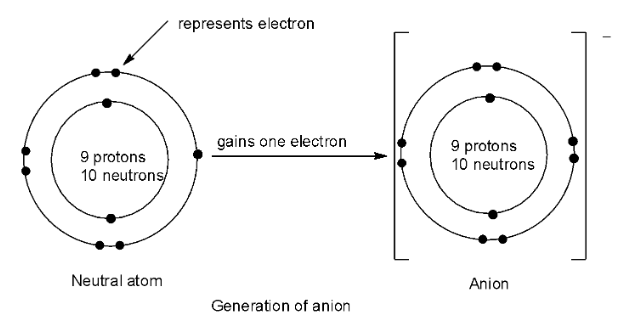QuestionAnswers

# Which of the following statements regarding an anion is not true?(A) The gain of an electron leads to the formation of an anion.(B) The radius of an anion is larger than the atomic radius of its parent atom.(C) The effective nuclear charge increases when an anion is formed.(D) Electron clouds expand due to increased repulsion among the electrons.

Hint: (1) An anion is attracted towards the anode in electrolysis and hence the name anion.
(2) Increase in the number of electrons leads to increase in the atomic size.
(3) Increase in the number of electrons in an atom leads to decrease in the effective nuclear charge experienced by the outermost electron.
(4) An electron cloud defines the region which has a high probability of containing electrons.

Complete step by step answer: An ion which results from an atom or molecule when it gains one or more valence electrons thereby giving a negative charge is called an anion. Thus, anions have more electrons than protons. Therefore, the statement A is true.The ionic radius of an ion is equal to the distance between the centre of the nucleus of the ion and its outermost shell containing electrons. When extra electrons are added, this distance will increase and hence the ionic radius of an anion will increase and will be larger than the parent atom. So, statement B is true.
Effective nuclear charge is the attractive force faced by the electron when it is shielded by the core electrons. When the number of electrons increases, the shielding of the outermost electron by the core electrons will increase and hence the nuclear charge experienced by it will be reduced. This happens in the case of an anion. So, statement C is not true.
Due to increase in electrons in an anion, the repulsion among the electrons increases leading to the increase in the size of the electron cloud and thus the electron cloud expands. So, the statement D is true.

So, thus option C is not true.

Note: The ion which is formed by the loss of electrons is known as cation. It has more protons and electrons and hence, it has positive charge. The ionic radius of the cation is smaller than the radius of the parent atom which also means that the radius of the cation is smaller than the radius of the corresponding anion. Moreover, the effective nuclear charge will increase when a cation is formed.